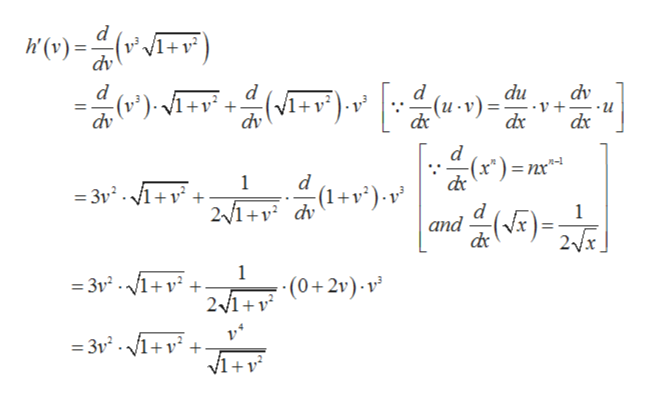# 2.5 Can you show me the steps to solve this?Directions: Find the derivative of the function.15) h(v) = v 3√1 +v^2

Question
9 views

2.5

Can you show me the steps to solve this?

Directions: Find the derivative of the function.

15) h(v) = v 3√1 +v^2

check_circle

Step 1

Given,

Step 2

Differentiating with res...help_outlineImage Transcriptionclosed (v) dv +v C)= dv d d + dv () d -(u v)= du - V + -u dv d d 1 = 3v. 1+v + 2/1+ dv )- v and 1 2x 1 3vV1 21 (2)- v =3v2.1+v fullscreen

### Want to see the full answer?

See Solution

#### Want to see this answer and more?

Solutions are written by subject experts who are available 24/7. Questions are typically answered within 1 hour.*

See Solution
*Response times may vary by subject and question.
Tagged in

### Calculus